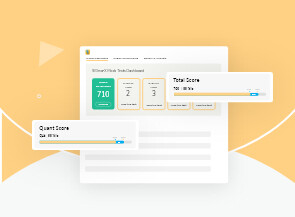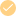Payal Tandon
Co-founder, e-GMAT
Welcome to e-GMAT Support!
I am Payal, Co-Founder of e-GMAT.
Feel free to ask any Query.We will be contacting you soon on

## OG 2020: Question No. 320

Is the average (arithmetic mean) of the numbers x, y, and z greater than z?

1. z − x < y − z
2. x < z < y

 Source OG 2020 Type Data Sufficiency Topic Algebra, Number Properties Sub-Topic Inequalities/ Statistics Difficulty Medium

### Solution

#### Steps 1 & 2: Understand Question and Draw Inferences

In this question, we are asked to determine whether the average (arithmetic mean) of the numbers x, y, and z is greater than the number z or not.

• If (x + y +z) / 3  > z

Then, x + y + z > 3z

Or, x + y > 2z

Hence, if the value of x + y is greater than 2z, then we can conclude that the average of x, y, and z is greater than z.

With this understanding, let us now analyse the individual statements.

#### Step 3: Analyse Statement 1

As per the information given in statement 1, z – x < y – z

• z – x < y – z

Or, x + y > z + z

Or, x + y > 2z

As we can derive that x + y > 2z, we can say the average of x, y, and z is greater than z.

Hence, statement 1 is sufficient to answer the question.

#### Step 4: Analyse Statement 2

As per the information given in statement 2, x < z < y.

• However, from this statement, we cannot say whether x + y > 2z or not.

Hence, statement 2 is not sufficient to answer the question.

#### Step 5: Combine Both Statements Together (If Needed)

Since we can determine the answer from statement 1 individually, this step is not required.

Hence, the correct answer choice is option A.

Did you know a 700+ GMAT Score can increase your chances to get into your dream business school? We can help you achieve that. Why don’t you try out our FREE Trial? We are the most-reviewed online GMAT Preparation company in GMATClub with more than 1950 reviews.#### Ashutosh

###### Don't limit yourself!# Take a free diagnosticSIGma-X mock test

##### Gauge your section-wise ability and get started with your GMAT prep!##### Adaptive mock test with ESR+ analysis##### 400+ Practice questions with detailed solutions##### 10+ hours of AI-driven video lessons
Take the first step to score 740+